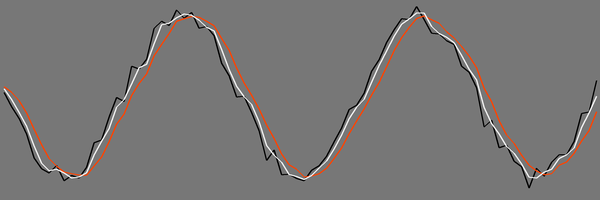# Quick Tip: Use the "Ring Buffer" Data Structure to Smooth Jittery Values

While developing a game, you might find values which are too noisy for your needs. The common case is analog user input (mouse, touch or joystick), but the noise might come from the game systems too, like physics or steering behaviors, where approximate solutions or noncontiguous changes result in noise. In this tutorial, you'll learn a simple way to smooth those noisy values. The code examples are in C#, but they are easy to adapt to any other language.

## Ring Buffer

The simplest way to smooth the varying value is to take a number of the past samples and average them. We'll be using a constant number of samples, so a fixed-size array is a natural and efficient choice for storing these. Then, to avoid shifting that array, we'll use a trick: the "ring buffer" data structure.

Let's start by defining the data to store in our utility class:

Here we have our samples buffer, the sum of the samples, and the last used index into the array. The constructor allocates the buffer array, sets lastIndex to zero, and calls the reset() method:

Here, we fill the buffer with the specified initial value, and set the sum to match it. This method can be used any time you need to restart the smoothing to avoid memory effects of the past samples.

Now, the main method: pushing a new value into our ring buffer:

Here, we overwrite the oldest sample at lastIndex with the new one, but before that we adjust the sum by subtracting the old sample and adding the new one.

Then, we advance lastIndex so that it points to the next sample (which is now the oldest one). But if we just advance lastIndex we'll run out of array in no time, so when it gets out of the array bound, we wrap it around to zero.

That's why it's a ring buffer. It's essentially the same as shifting the array and appending the new sample, but much faster since instead of copying the values in memory we just wrap around the index.

Now, the only thing missing is getting the smoothed value:

That's it; we just divide the sum by the number of samples to get the average. If we didn't store the sum, we'd have to compute it here from the samples.

## Results

Let's take a look at the results:The black line is the original signal (sine wave with some noise), the white line is smoothed using two samples, and the red line is smoothed using four samples.

As you see, even a few samples make it noticeably smoother, but the more samples we use, the more it lags behind the original signal. That's expected since we only use past samples in the real-time case. If you're post-processing, you can shift the smoothed values in time to avoid the lag.

## Conclusion

You now have a simple utility class which can be used to smooth any noisy incoming values, whether it's user input, an object trail, or a speed indicator.

It can be further enhanced by adding sample weights (we used a simple average with constant 1/N weight), but that's a huge topic, digital signal processing, and better left to a future tutorial!